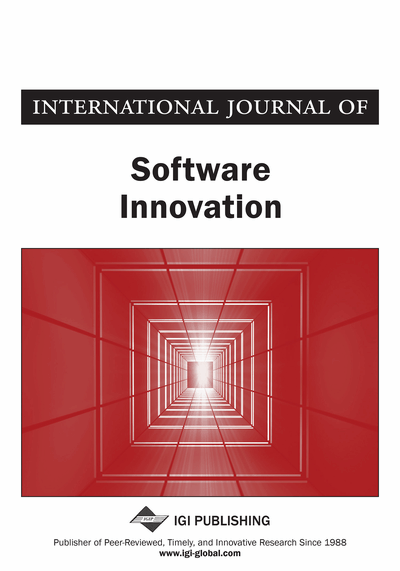# How to Systematically Embed Cycles in Balanced Hypercubes

Hsuan-Han Chang (Department of Computer Science and Information Engineering, National Dong Hwa University, Shoufeng, Taiwan), Kuan-Ting Chen (Department of Computer Science and Information Engineering, National Dong Hwa University, Shoufeng, Taiwan) and Pao-Lien Lai (Department of Computer Science and Information Engineering, National Dong Hwa University, Shoufeng, Taiwan)
DOI: 10.4018/IJSI.2017010104

## Abstract

The balanced hypercube is a variant of the hypercube structure and has desirable properties like connectivity, regularity, and symmetry. The cycle is a popular interconnection topology and has been widely used in distributed-memory parallel computers. Moreover, parallel algorithms of cycles have been extensively developed and used. The problem of how to embed cycles into a host graph has attracted a great attention in recent years. However, there is no systematic method proposed to generate the desired cycles in balanced hypercubes. In this paper, the authors develop systematic linear time algorithm to construct cycles and Hamiltonian cycles for the balanced hypercube.
Article Preview
Top

## 1. Introduction

The balanced hypercube, a variation of the hypercube with better properties than the hypercube with the same number of vertices, was proposed by Wu and Huang (1997). The balanced hypercube is an edge transitive and vertex transitive graph. More desirable properties of balanced hypercubes were studied. This topic has been covered many times in past studies. Huang and Wu (1995) have shown that the balanced hypercube can be implemented at least as efficient as the standard hypercube in an area layout and more efficient in a linear layout. Huang and Wu (1997) have studied the resource placement problem in balanced hypercubes. Xu, Hu, and Xu (2007) have proved that the balanced hypercubes is Hamiltonian laceable. Yang (2010) has proposed that the balanced hypercube is edge-bipancyclic and Hamiltonian laceable.

A path or cycle structure is a fundamental network for multiprocessor systems and suitable for developing simple algorithms with low communication costs. Several efficient algorithms have been designed with respect to cycle structures for solving a variety of algebraic problems, graph problems, and some parallel applications, such as those in image and signal processing (Akl 1997). In the article by Baoyuan and Yizhou, the authors have studied efficient GPU-based parallel algorithms for H-tree based data cube operations. Jianxin, Qilong and Jianer (2011) have studied the k-path problem. Leighton (1992) has proposed some parallel algorithms and Architectures.

To carry out a cycle structure algorithm on a multiprocessor computer, the processes of the parallel algorithm need to be mapped to the vertices of the interconnection network in the system so any two adjacent processes in the cycle are mapped to two adjacent vertices of the network. With regard to cycle embedding of interconnection networks, many interesting results have been obtained (Chang, Sung, & Hsu, 2000; Cheng, Hao, & Feng, 2014; Chen, Lai, & Tsai, 2010; Chen, Hsu, & Tan, 2006; Fan, Lin, & Jia, 2005; Fan, Jia, & Lin, 2008; Hao, Zhang, Feng, & Zhou, 2014; Kueng, Lin, Liang et al., 2008; Li, Peng, & Chu, 2002; Wang, 2008).

In particular, Zheng and Latifi (1997) introduced the notion of the reflected edge label sequences and proposed a kind of codeword, termed the generalized Gray code. In this paper, we extend the concepts of reflected edge label sequences and cycle patterns in Chen et al. (2010), Zheng and Latifi (1997), then propose, efficient algorithms for embedding cycles in balanced hypercubes.

The rest of this paper is organized as follows. First, we present the background and definitions relating to the balanced hypercube in Section 2. In Section 3, we propose alternated edge label sequence, reflected edge label sequence, double reflected edge label sequence, complete reflected edge label sequence, and independent alternated permutation, then demonstrate how to embed cycles employing each in the balanced hypercube. Finally, we propose zero alternated permutation and study how to construct Hamiltonian cycles using it in Section 4. The conclusion is given in the final section.

## Complete Article List

Search this Journal:
Reset
Open Access Articles: Forthcoming
Volume 9: 4 Issues (2021): Forthcoming, Available for Pre-Order
Volume 8: 4 Issues (2020)
Volume 7: 4 Issues (2019)
Volume 6: 4 Issues (2018)
Volume 5: 4 Issues (2017)
Volume 4: 4 Issues (2016)
Volume 3: 4 Issues (2015)
Volume 2: 4 Issues (2014)
Volume 1: 4 Issues (2013)
View Complete Journal Contents Listing